Select Page

# Maths Application of Integrals CBSE 12 Science MCQ in English Answers

Maths Application of Integrals CBSE 12 Science MCQ in English Answers to enable students to get Answers in a narrative video format for the specific question.

Expert Teacher provides Maths Application of Integrals CBSE 12 Science MCQ English Answers. This video solution will be useful for students to understand how to write an answer in exam in order to score more marks. This teacher uses a narrative style for a question from Application of Integrals not only to explain the proper method of answering question, but deriving right answer too.

Please find the question below and view the Answer in a narrative video format.

Question:

## Similar Questions from CBSE, 12th Science, Maths, Application of Integrals

Question 1 : Find the area of the region bounded by the parabola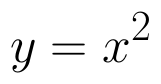and y = | x |. (View Answer Video)

Question 2 : Using integration find the area of the triangular region whose sides have the equations y = 2x + 1, y = 3x + 1 and x = 4. (View Answer Video)

Question 3 : Find the area bounded by the curve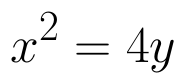and the line x = 4y - 2. (View Answer Video)

Question 4 : Using the method of integration, find the area of the region bounded by the lines 3x - y - 3 = 0, 2x + y - 12 = 0 and x -2y - 1 = 0. (View Answer Video)

Question 5 : Find the area of the region bounded by the curve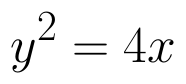and. (View Answer Video)

### Three Dimensional Geometry

Question 1 : Find the distance of the point (1, -2, 3) from the plane x-y+z=5 measured parallel to the line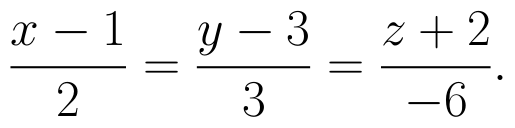(View Answer Video)

Question 2 : If lines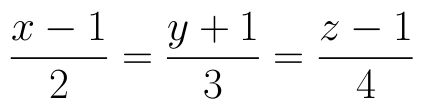and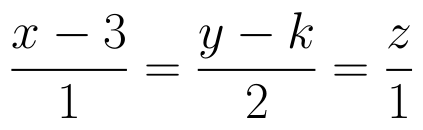intersect, then find the value of k and hence find the equation of plane containing these lines. (View Answer Video)

Question 3 : Find the distance of point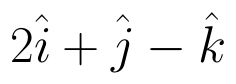from the  plane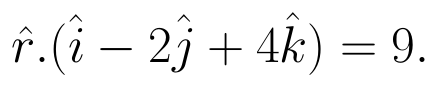(View Answer Video)

Question 4 : Find the vector equation of a plane which is at a distance of 5 units from the origin and its normal vector is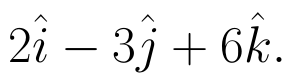(View Answer Video)

Question 5 : A plane makes intercepts -6, 3, 4 respectively on the co-ordinte axes. Find the length of the perpendicular from the origin on it. (View Answer Video)

### Relations and Functions

Question 1 : Let * be the binary operation on N given by a * b = LCM of a and b. Find the identity of * in N. (View Answer Video)

Question 2 : Number of binary operations on the set { a, b} are: (View Answer Video)

Question 3 :  Letdefined as f(x) = 5 be a constant function. Then its range is (View Answer Video)

Question 4 : If f is the greatest integer function and g is the modulus function . Write the value of g o f(-1/3) – f o g ( -1/3 ). (View Answer Video)

Question 5 : If the mapping f and g are given by f = {(1, 2), (3, 5), (4, 1)} and g = {(2, 3), (5, 1), (1, 3)}, Find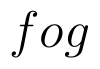. (View Answer Video)

### Linear Programming

Question 1 : The objective function is maximum or minimum, which lies on the boundary of the feasible region. (View Answer Video)